# Theory

The “Kneedle” algorithm has been published by Satopää, Albrecht, Irwin, and Raghavan  using the concept of curvature as a mathematical measure of much a function differs from a straight line. In consequence, the maximum curvature captures the levelling off effects operators use to identify knees.

For continuous functions, the curvature is described as shown here:

Let’s create for instance N = 10,000 random standard normal distributed data points and display them in a histogram: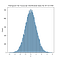Random standard normal distributed data plotted in a histogram with Kernel Density Estimation (Image by author)

Now we can sort the values in an ascending order to get a cumulative distribution function (CDF) showing each x-value on the x-axis and its probability of occurrence on the y-axis: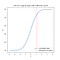Sorted data displayed as CDF with maximum curvature indicated at x = 1.16 (Image by author, inspired by Figure 1 in Satopää et al., 2011 )

While curvature is well defined for continuous functions, there is no clear definition of curvature for discrete data. In this instance, the curvature can be fitted to a continuous function — but when the data is noisy, this fitting can become even more difficult. This is where the “Kneedle” algorithm comes into play.

Let’s take a deeper look how this algorithm works. For this, I have used the original discrete data set from Figure 2 from the conference paper from Satopää et alia . It’s provided by the Python package “kneed”:

`import kneedkneed.DataGenerator.figure2()`

This is the raw data being plotted:

Let’s get through the steps the “Kneedle” algorithm follows:

1. Smoothing spline

Using a spline to “smooth” the shape of the raw data.

2. Normalizing to the unit square

Range of x- and y-values will be normalized to 0 to 1 (see axes).

3. Calculating the difference curve changes

Calculating perpendicular distances (Euclidean distances from the data points to diagonal given line from first to last data point)…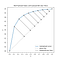Perpendicular lines indicating perpendicular distances (Image by author, inspired by Figure 2a in Satopää et al., 2011 )

… and rotating them by 45 degrees counter clockwise. The magnitude of these rotated perpendicular lines indicates the difference values.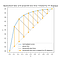Perpendicular lines rotated by 45 degrees representing difference values (Image by author, inspired by Figure 2b in Satopää et al., 2011 )

4. Calculating the local maxima of the difference curve

Finding the “knee” points at the local maxima of the difference curve in the normalized curve (for detecting elbow points, the graph will be inverted).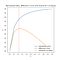Maximum curvature at x = 0.22 (Image by author, inspired by Figure 2c in Satopää et al., 2011 )

5. Calculating threshold for each local maximum in the difference curve

For each local maximum in the difference curve, there is a unique threshold value that is based on the average difference between the x-values and a sensitivity parameter (please see Satopää et al., 2011  for further reference how this threshold is computed in detail). This sensitivity parameter measures how many “flat” points are expected to be seen in the original unmodified data before declaring a “knee” and can be adjusted: A small sensitivity value detects knees quicker, whereas a larger one is more conservative.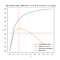Threshold indicated at y = 0.43 with a sensitivity of 1 (Image by author, inspired by Figure 2c in Satopää et al., 2011 )

6. Each difference value is compared with threshold

If a difference value drops below the threshold before the local maximum is reached, the algorithm is declaring a “knee”. Conversely, the threshold value is reset to zero.

## kneed

With this, we can for instance directly plot the normalized difference curve including the “knee” point:

We can use the method KneedLocator() in order to find a knee/elbow in our data:

`# calculate and show knee/elbowkneedle = kneed.KneeLocator(…)knee_point = kneedle.knee #elbow_point = kneedle.elbowprint('Knee: ', knee_point) #print('Elbow: ', elbow_point)kneedle.plot_knee()`

These are the most important parameters for KneedLocator():

• curve: ”concave” for a “knee”, convex for an “elbow” — based on the negative/positive concavity of the function
• direction: “increasing” for a positive slope, “decreasing” for a negative slope of the function
• online: Knee as first element detected (False) or correcting “old” knee values if necessary if points are received (True)
• S: Sensitivity for knee detection (S=0 or bigger); Satopää et alia  state that “kneedle” has perfect information in offline setting when sensitivity is 0 whereas in online settings, overall a sensitivity of 1 shows the best overall performance (but it can vary from the data points received).

# Applications

## Clustering

a) K-Means

With the elbow method, you can calculate the within-cluster sum of squares as a measure of the within-cluster variance and detect the best value for the number of clusters to form (k):

b) DBSCAN

When calculating the distance metric (usually Euclidean distance) for each data point and sorting them in an ascending order, they can be plotted in a k-distances graph to a find a threshold value for defining a cluster:

# Final remarks

https://share.streamlit.io/arvkevi/ikneed/main/ikneed.py

In my opinion, “kneed” is currently the most powerful Python package for knee detection as it provides a lot of useful functions. Nevertheless, there are other similar Python packages provided for finding knees of a curve, such as “kneebow”:

# References

 V. Satopää, J. Albrecht, D. Irwin and B. Raghavan, “Finding a “Kneedle” in a Haystack: Detecting Knee Points in System Behavior” (2011), 31st International Conference on Distributed Computing Systems Workshops, 2011, pp. 166–171, doi: 10.1109/ICDCSW.2011.20.

Industrial and organizational psychologist specialized in the fields of Data Analytics and Data Science with a focus on Machine Learning

## More from Daniel Kleine

Industrial and organizational psychologist specialized in the fields of Data Analytics and Data Science with a focus on Machine Learning